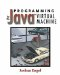# 13.3 Unification

The key to a Prolog implementation is unification. Two things unify if there is a substitution of the variables so that they are equal. For example, consider the question

` ?- inside(baltimore, X). `

What Prolog does is to look at all its rules and see whether there is a way to substitute for X such that X is identical to one of its rules. Prolog was given this rule:

` inside(baltimore, maryland). `

It's easy to see that if X is maryland, then the two become identical. Thus Prolog reports,

` Yes, X=maryland `

#### 13.3.1 Unification Algorithm

The code to handle unification is kept in a class called Prolog. This method implements unification:

` public static boolean unify(Object x, Object y) {    x = deref(x);    y = deref(y);        if(x.equals(y))         return true;    if(x instanceof Var) {         setBinding((Var) x, y);         return true;    }    if(y instanceof Var) {         setBinding((Var) y, x);         return true;    }    return false; } `

The unification algorithm begins by dereferencing x and y. Dereferencing is the process of substituting a variable's binding for the variable itself. This ensures that the variable is used consistently in all cases.

Dereferencing is performed by the method deref, which checks to see whether its argument is a bound variable; if so, it returns the current binding of that variable. The variable may be bound to another variable, so deref repeats the procedure until the variable is completely dereferenced. Constants and unbound variables dereference to themselves. Here is the code for deref:

` public static Object deref(Object x) {    while(x instanceof Var && ((Var) x).binding != null)        x = ((Var) x).binding;    return x; } `

After dereferencing both values, unify checks to see whether they are equal. If they are equal, then they unify without doing any additional work and unify returns true.

If x is a variable, then it can be unified with y by setting the binding of x to y; the reverse is true for y. The binding is done using setBinding, which is discussed in the next section. Then unify returns true.

If x and y are not equal and neither x nor y is a variable, then they do not unify. In that case, unify returns false.

#### 13.3.2 Managing Bindings

The method setBinding sets the binding of a variable to a value. The code for setBinding is

` public static void setBinding(Var var, Object val) {    var.binding = val;    trail.push(var); } `

Setting var's binding field to val binds var to val.

This Prolog implementation makes use of a stack called the trail to keep track of variable bindings. Whenever Prolog binds a variable to a value, it pushes the variable onto the trail. The trail is represented using the Stack class in java.util:

` static Stack trail = new Stack(); `

During backtracking, any variables bound during the code being backtracked over must become unbound. This allows later code to establish new bindings for these variables. This is done by a method called undoBindings:

` static void undoBindings(Object token) {    Object var;    while((var = trail.pop()) != token)        if(var instanceof Var)             ((Var) v).binding = null; } `

The method undoBindings searches for a token that marks some point in the stack. It unbinds all variables until it finds the token.

The token is used within the code implementing a rule. The code pushes a new token onto the stack when it begins code that it will backtrack over. All bindings on the stack below the token should not be touched; all bindings above the token are unbound. To obtain a token and push it onto the stack, the rule uses markTrail:

` static Object markTrail() {    Object token = new Object();    trail.push(token);    return token; } `

The caller must remember the token for later use with undoBindings.

undoBindings repeatedly pops the stack looking for the token. Until it finds it, it unbinds all the Vars it finds on the stack by setting its binding to null.Programming for the Javaв„ў Virtual Machine
ISBN: 0201309726
EAN: 2147483647
Year: 1998
Pages: 158
Authors: Joshua Engel

Similar book on Amazon